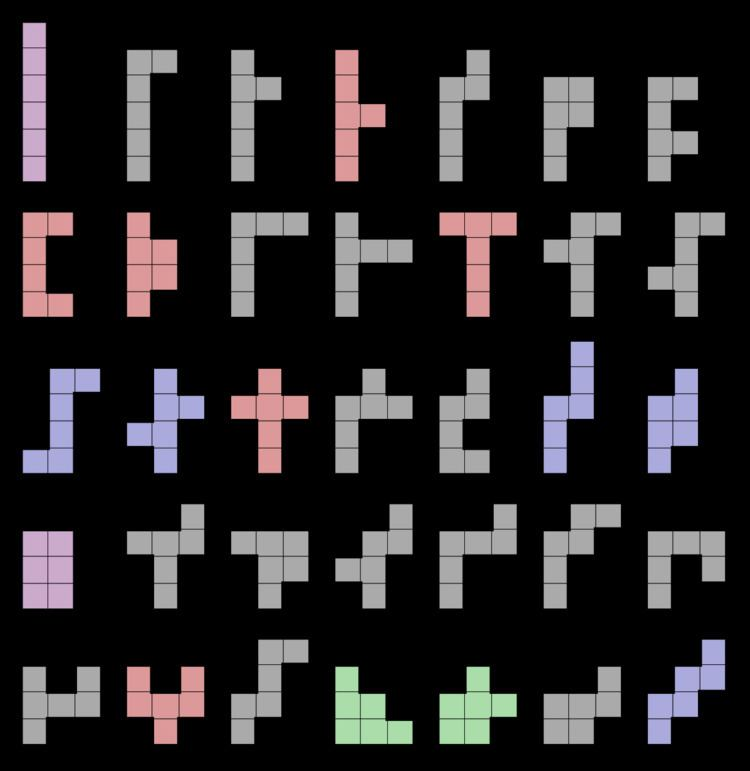# Hexomino

Updated onA hexomino (or 6-omino) is a polyomino of order 6, that is, a polygon in the plane made of 6 equal-sized squares connected edge-to-edge. The name of this type of figure is formed with the prefix hex(a)-. When rotations and reflections are not considered to be distinct shapes, there are 35 different Free hexominoes. When reflections are considered distinct, there are 60 one-sided hexominoes. When rotations are also considered distinct, there are 216 fixed hexominoes.

## Symmetry

The figure shows all possible free hexominoes, coloured according to their symmetry groups:

• 20 hexominoes (coloured grey) have no symmetry. Their symmetry group consists only of the identity mapping.
• 6 hexominoes (coloured red) have an axis of mirror symmetry parallel to the gridlines. Their symmetry group has two elements, the identity and a reflection in a line parallel to the sides of the squares.
• 2 hexominoes (coloured green) have an axis of mirror symmetry at 45° to the gridlines. Their symmetry group has two elements, the identity and a diagonal reflection.
• 5 hexominoes (coloured blue) have point symmetry, also known as rotational symmetry of order 2. Their symmetry group has two elements, the identity and the 180° rotation.
• 2 hexominoes (coloured purple) have two axes of mirror symmetry, both parallel to the gridlines (thus one horizontal axis and one vertical axis). Their symmetry group has four elements. It is the dihedral group of order 2, also known as the Klein four-group.
• If reflections of a hexomino are considered distinct, as they are with one-sided hexominoes, then the first and fourth categories above would each double in size, resulting in an extra 25 hexominoes for a total of 60. If rotations are also considered distinct, then the hexominoes from the first category count eightfold, the ones from the next three categories count fourfold, and the ones from the last category count twice. This results in 20 × 8 + (6+2+5) × 4 + 2 × 2 = 216 fixed hexominoes.

## Packing and tiling

Each of the 35 hexominoes satisfies the Conway criterion; hence every hexomino is capable of tiling the plane.

Although a complete set of 35 hexominoes has a total of 210 squares, it is not possible to pack them into a rectangle. (Such an arrangement is possible with the 12 pentominoes which can be packed into any of the rectangles 3 × 20, 4 × 15, 5 × 12 and 6 × 10.) A simple way to demonstrate that such a packing of hexominoes is not possible is via a parity argument. If the hexominoes are placed on a checkerboard pattern, then 11 of the hexominoes will cover an even number of black squares (either 2 white and 4 black or vice versa) and 24 of the hexominoes will cover an odd number of black squares (3 white and 3 black). Overall, an even number of black squares will be covered in any arrangement. However, any rectangle of 210 squares will have 105 black squares and 105 white squares.

However, there are other simple figures of 210 squares that can be packed with the hexominoes. For example, a 15 × 15 square with a 3 × 5 rectangle removed from the centre has 210 squares. With checkerboard colouring, it has 106 white and 104 black squares (or vice versa), so parity does not prevent a packing, and a packing is indeed possible. It is also possible for two sets of pieces to fit a rectangle of size 420, or for the set of 60 one-sided hexominoes (18 of which cover an even number of black squares) to fit a rectangle of size 360.

## Polyhedral nets for the cube

A polyhedral net for the cube is necessarily a hexomino, with 11 hexominoes actually being nets. They appear on the right, again coloured according to their symmetry groups.

## References

Similar Topics
Fools for Luck
Harry Aikines Aryeetey
Jean Luc Migué
Topics